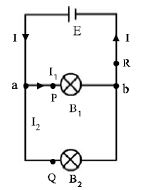Physics

# What is Parallel Circuit?The circuit in which the electric components are arranged in such a way that one terminal of all the components are joined at a common point and the other terminal are joined at another common point then this circuit is called a parallel circuit. In figure, one end of the bulbs B1 and B2 are connected at the point and the other end at the point b and so formed a parallel circuit. In a parallel circuit there are alternative paths for the current to flow.Say, the total current in the circuit is I which splits into two parts I1 and I2 at the junction a. Let I1 and I2 are the currents flowing through the bulbs B1 and B2 respectively. At the junction b the currents I1 and I2 recombine to form the current I again. If the current at the points P, Q and R is measured by an ammeter, then it will be found that

I = I1 + I2

Here, total current of the circuit = I

i.e. in a parallel circuit, the sum of the individual currents flowing through each of the parallel branches is equal to the total current.

The electrical appliances such as- light, fan etc. which we use in houses or offices is connected in parallel to the AC mains. Each of the appliances gets the same voltage supply due to parallel connection. But they get different amounts of current.